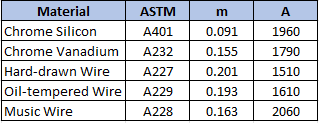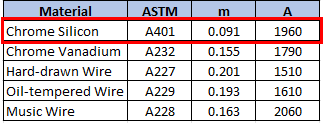## Chrome Silicon Wire

A chrome silicon wire with a diameter of 10 cm will be used as a spring material in a static assembly. Approximate the max allowable torsional stress for the wire.Hint
The max allowable torsional stress for hardened and tempered carbon and low-alloy steels in static applications can be approximated as:
$$S_{sy}=\tau = 0.50\times S_{ut}$$$Hint 2 For spring materials, the minimum tensile strength for common spring steels can be determined from: $$S_{ut}=\frac{A}{d^{m}}$$$
where $$S_{ut}$$ is the tensile strength in MPa, and $$d$$ is the wire diameter in millimeters.
The max allowable torsional stress for hardened and tempered carbon and low-alloy steels (ASTM A232 & A401) in static applications can be approximated as:
$$S_{sy}=\tau = 0.50\times S_{ut}$$$For spring materials, the minimum tensile strength for common spring steels can be determined from: $$S_{ut}=\frac{A}{d^{m}}$$$
where $$S_{ut}$$ is the tensile strength in MPa, $$d$$ is the wire diameter in millimeters, and $$A$$ and $$m$$ can be found in the below table:Since the problem is asking for a chrome silicon wire:
$$S_{ut}=\frac{1960}{(100mm)^{0.091}}=\frac{1960}{1.521}=1,289\: MPa$$$Thus, the max allowable torsional stress for a chrome silicon wire is approximately: $$\tau = 0.50\times (1,289MPa)=645\:MPa$$$
645 MPa